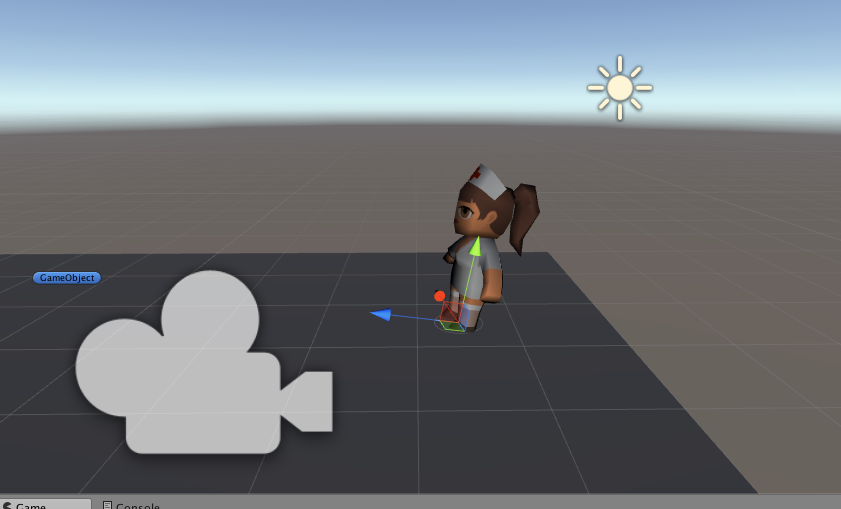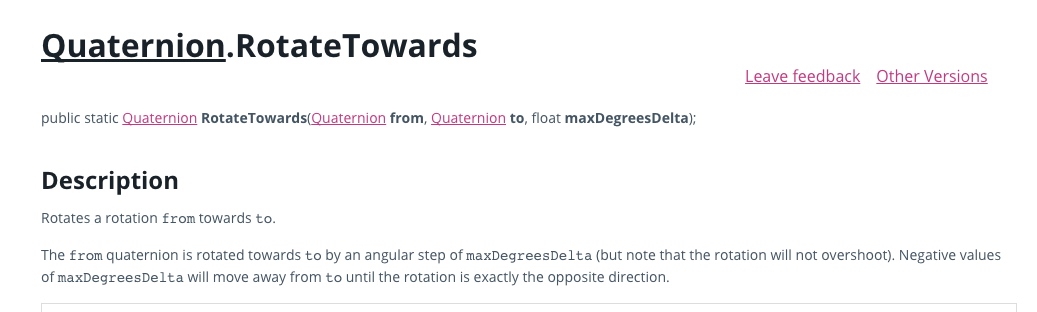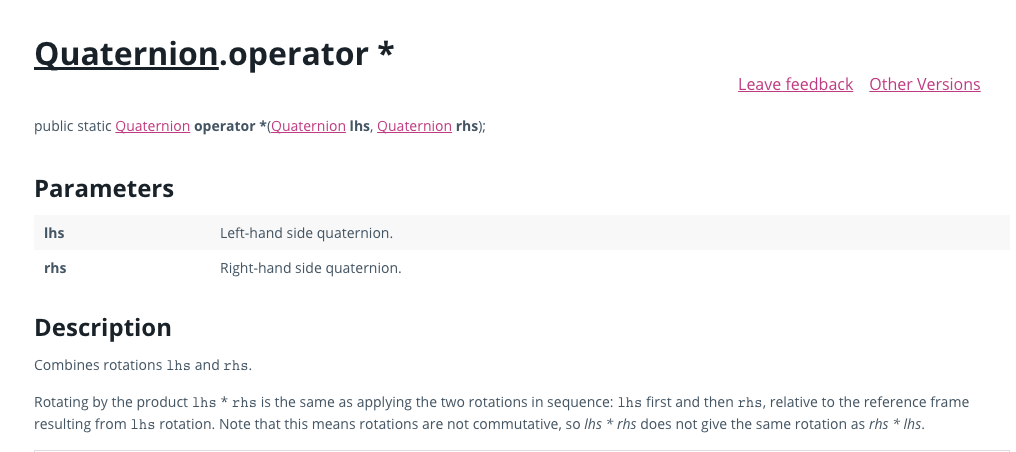# unity初识之Quaternion和旋转

#### 与之前做2d游戏不一样，3d游戏中，角度变换，用四元数来表示和计算

unity对于四元数的定义

### - 矩阵形式。

 缺点是：
矩阵占据更多内存。3x3矩阵，就是9个数，代表一个方位，如果是动画的话，一帧中如果做旋转操作，并且算上骨骼旋转，皮肤旋转，量很大，何况一个动画会有很多帧。
难于使用。矩阵是用的向量，都是-1到1之间的值，人类平时描述都是角度。
矩阵可能是病态的，可能有冗余。


### - 欧拉角形式。

 基本思想是将角位移分解为绕三个互相垂直轴的三个旋转组成的序列。用欧拉角是有顺序的，比如untiy中，x,y,z分别为right,up，forward 。  也就是比如旋转一个x , y ,z都转的角度，是先转一个，再转一个，再转最后一个。如果顺序改变，有可能最后的结果是不一样的(例子******)。     具体欧拉角顺序是什么，有个约定叫 “heading,pich,bank”，也就是Untiy中对应的约定，heading 也就是up,y轴，    pich就是right  x轴，   bank就是 forward,z轴。
![这里写图片描述](https://img-blog.csdn.net/20180302193130776?watermark/2/text/aHR0cDovL2Jsb2cuY3Nkbi5uZXQvcTI3MDI3NDk3OA==/font/5a6L5L2T/fontsize/400/fill/I0JBQkFCMA==/dissolve/70)
优点:
使用容易，使用的参数全都是角度的形式。在cocos和egret这些2d引擎中，之前做2d游戏，设置rotation也是直接就用的角度。
最简洁的表达。3d中描述一个方位，不能少于3个数，欧拉角只有3个。
任意三个数都是合法的。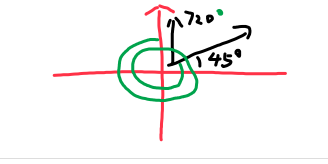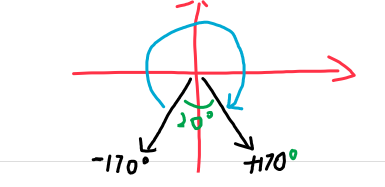### - 四元数形式

[w, v] , [w,(x,y,z)]

 优点:
平滑差值: 用slerp可以得到平滑的转角度的差值
快速连接和角位移求逆，四元数叉乘能将角位移序列转换成单个角位移。
能和矩阵快速转换。
仅用4个数。比矩阵强点，比欧拉角多一个。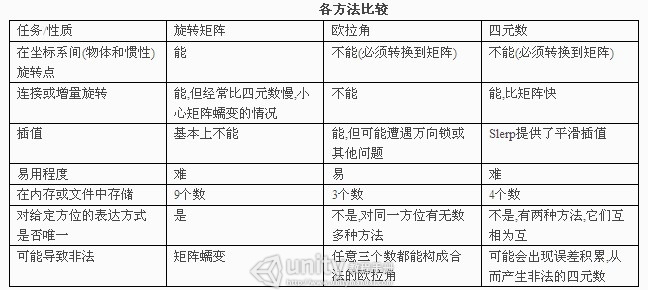Unity中 Quaternion类的理解与使用
https://docs.unity3d.com/ScriptReference/Quaternion.html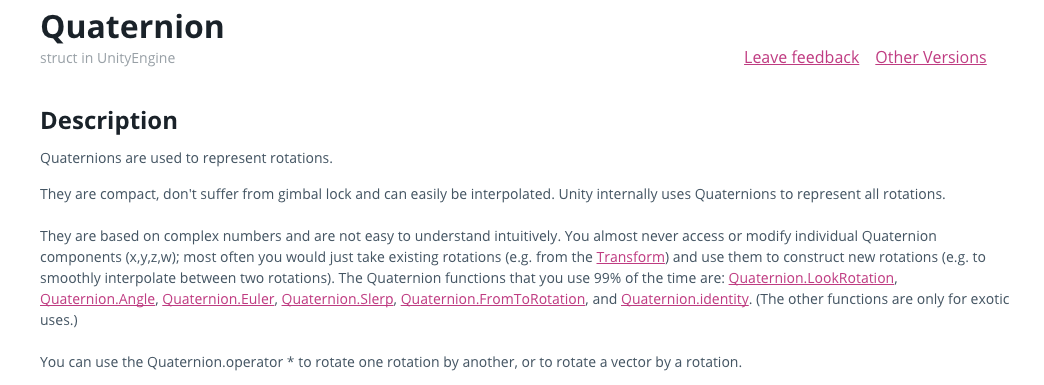#### 下面就挨个把各个工具函数的用法以及变现结果试一遍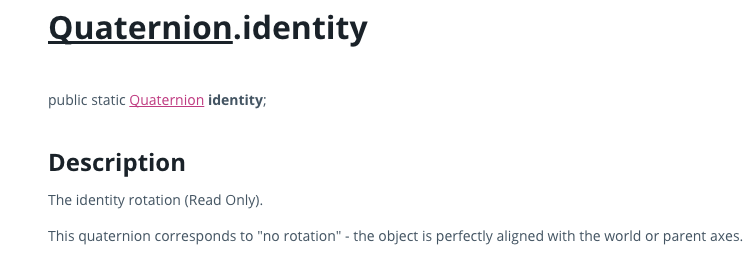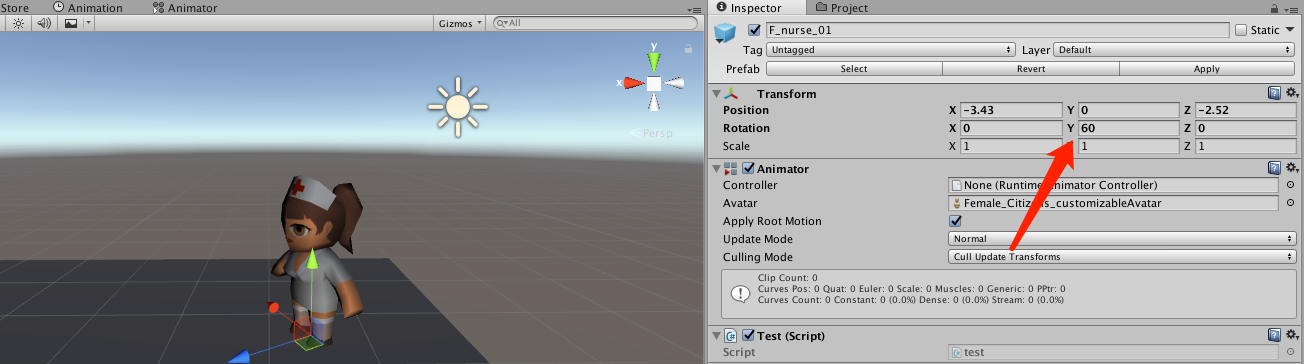public class ExampleClass : MonoBehaviour {
void Example() {
transform.rotation = Quaternion.identity;
}
}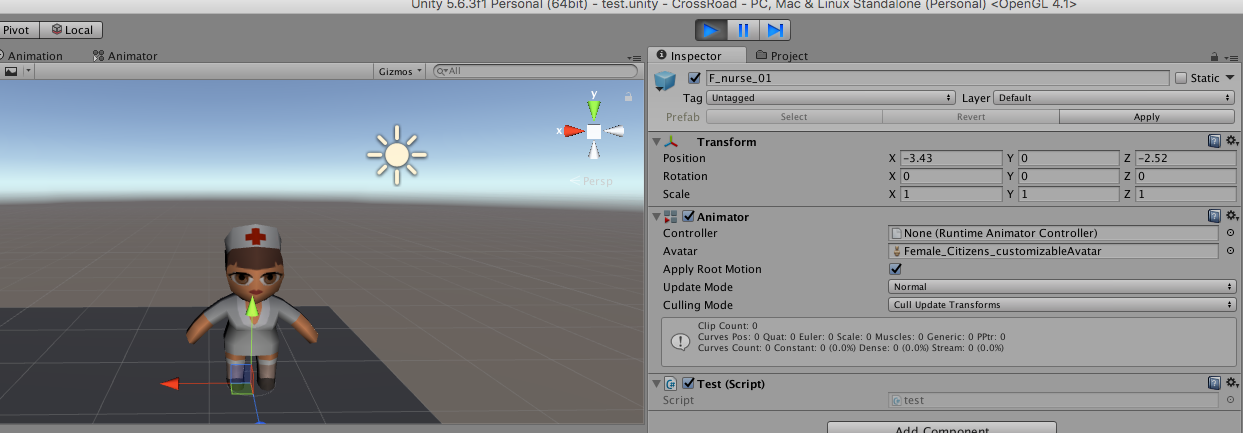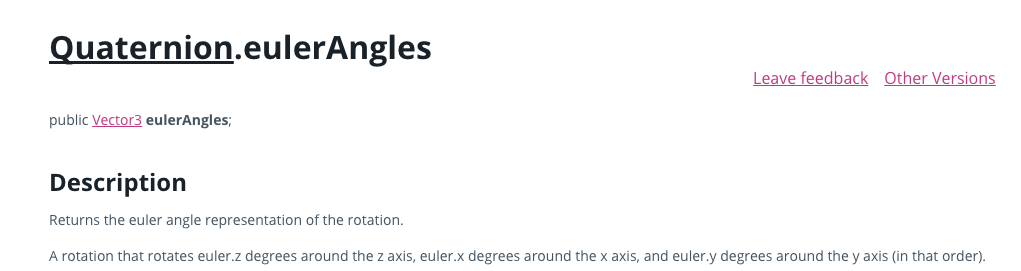public Quaternion rotation = Quaternion.identity;
void Example() {
rotation.eulerAngles = new Vector3(0, 30, 0);
print(rotation.eulerAngles);
}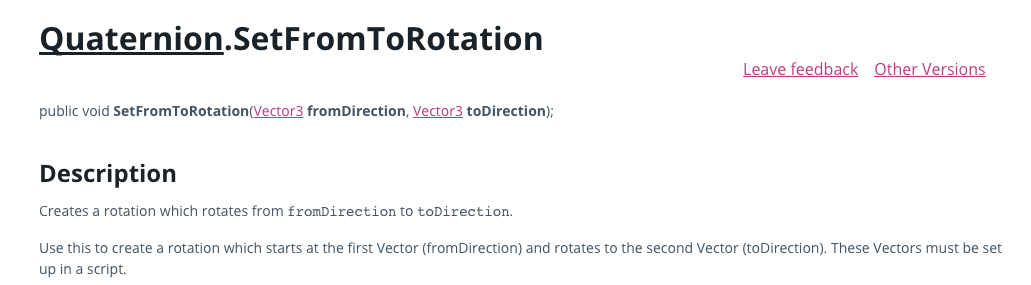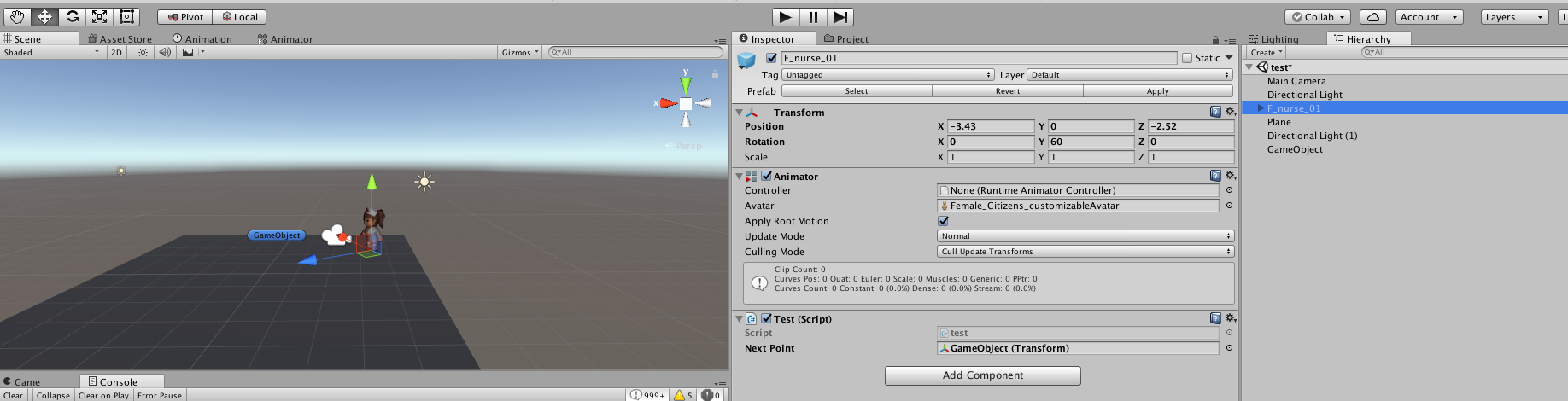//This is the Transform of the second GameObject
public Transform m_NextPoint;
Quaternion m_MyQuaternion;
float m_Speed = 1.0f;

void Start()
{
m_MyQuaternion = new Quaternion();
}

void Update()
{
//Set the Quaternion rotation from the GameObject's position to the next GameObject's position
m_MyQuaternion.SetFromToRotation(transform.position, m_NextPoint.position);
//Move the GameObject towards the second GameObject
transform.position = Vector3.Lerp(transform.position, m_NextPoint.position, m_Speed * Time.deltaTime);
//Rotate the GameObject towards the second GameObject

transform.rotation = m_MyQuaternion * transform.rotation;

Debug.Log(transform.rotation.eulerAngles);
}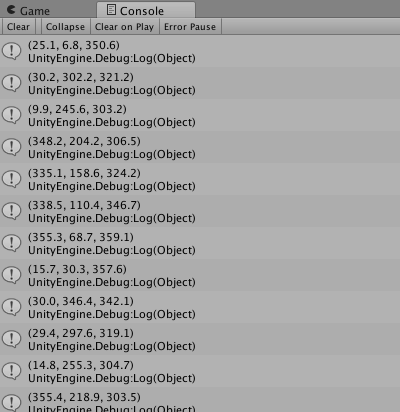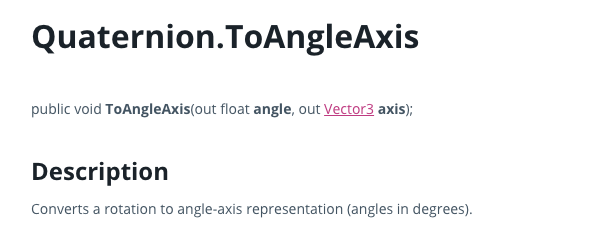unity editor中预设值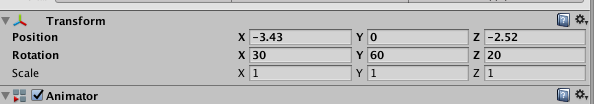public float angle = 0.0F;
public Vector3 axis = Vector3.zero;
void Example() {
transform.rotation.ToAngleAxis(out angle, out axis);
Debug.Log(" angle is  " + angle);

Debug.Log(" axis is  " + axis);
}

angle is 64.38145 axis is (0.6, 0.8, 0.0) 还没明白为啥。。。不过也不会常用吧。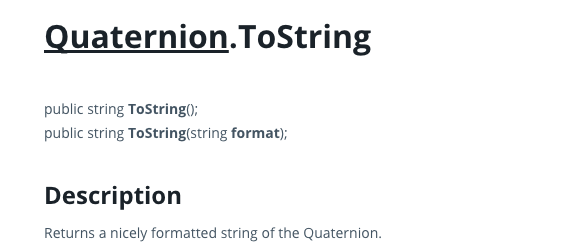print(Quaternion.identity);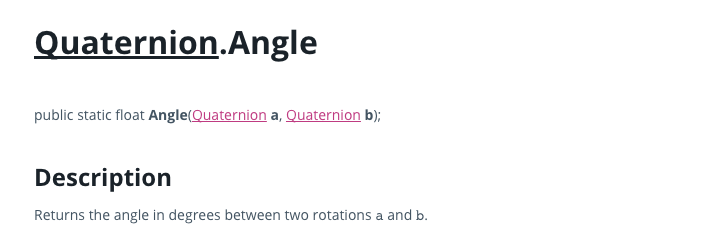public Transform target;
void Update() {
float angle = Quaternion.Angle(transform.rotation, target.rotation);
Debug.Log(angle);
}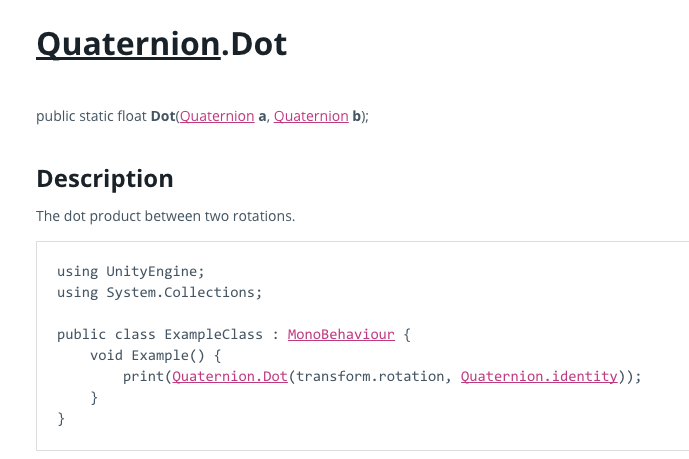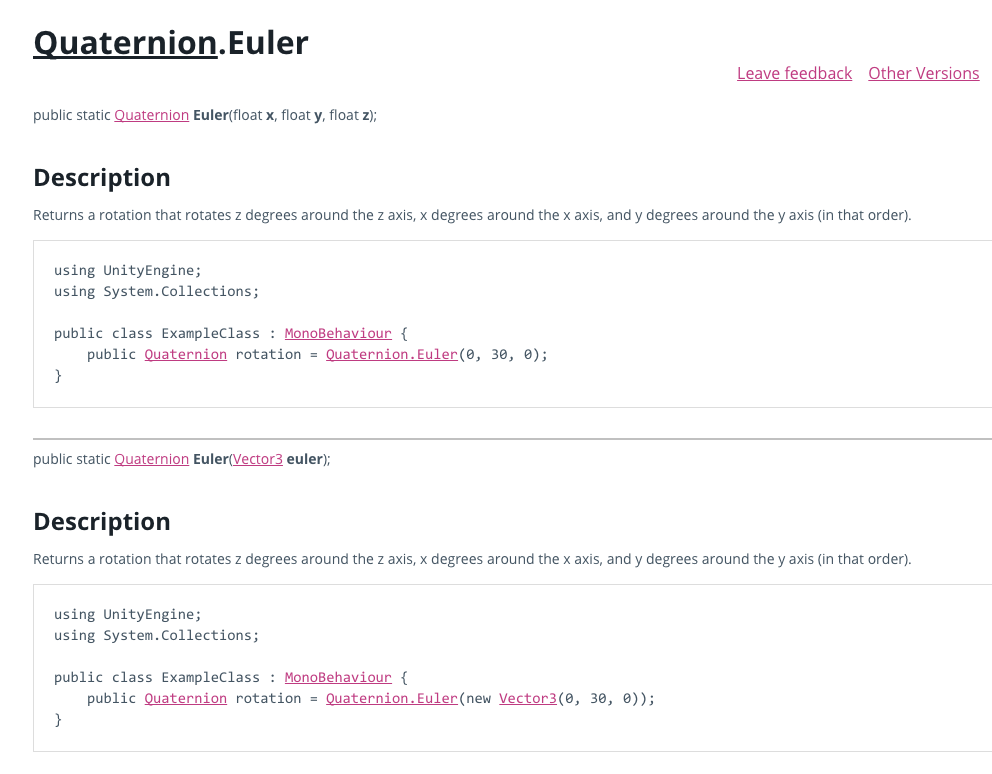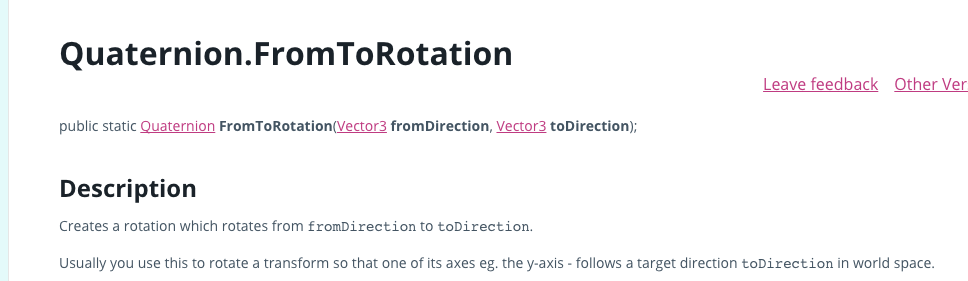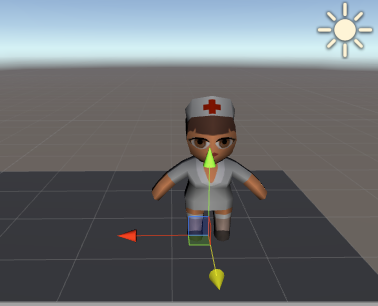void Awake() {
print(transform.forward);
transform.rotation = Quaternion.FromToRotation(Vector3.up, transform.forward);
print(transform.rotation.eulerAngles);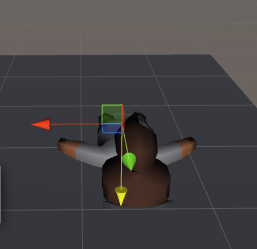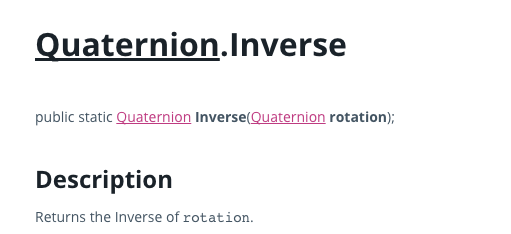void Awake() {
transform.rotation = Quaternion.Inverse(transform.rotation);
print(transform.rotation.eulerAngles);
}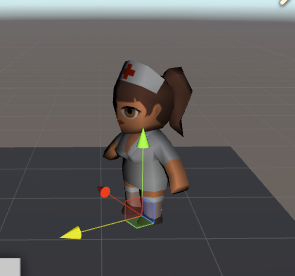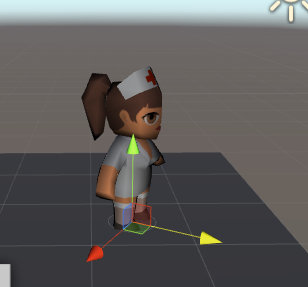inverse之后是 (0,300,0) 也就是 (0,-60,0)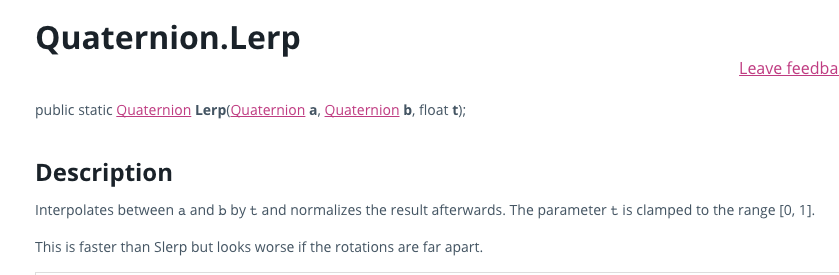lerp比slerp快 但是如果旋转角度离得远看起来会很糟糕。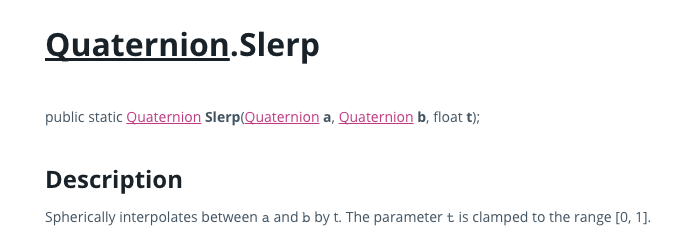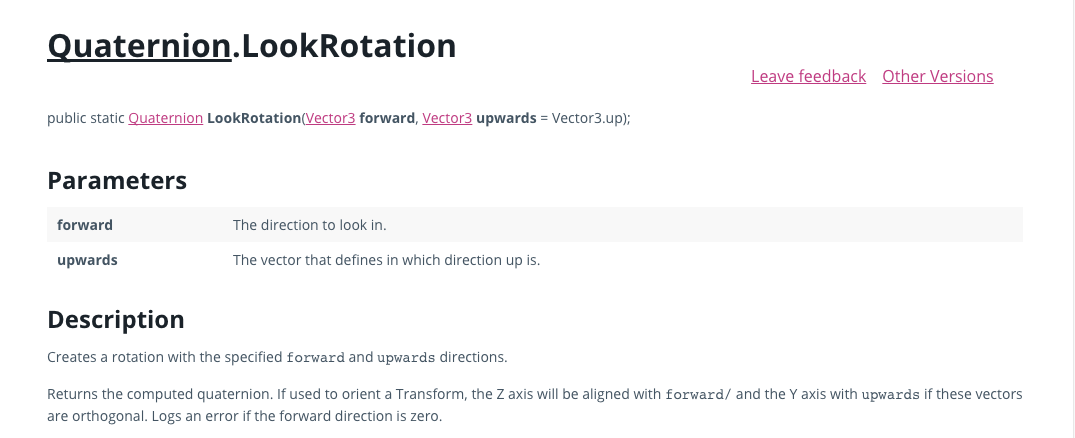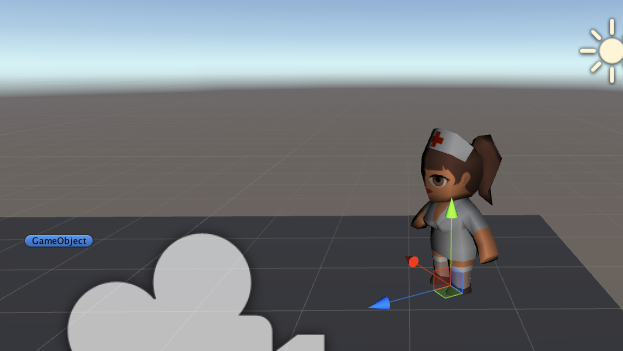public Transform to;
void Awake() {
Vector3 relativePos = to.position - transform.position;
Quaternion rotation = Quaternion.LookRotation(relativePos);
transform.rotation = rotation;
Debug.Log(transform.rotation.eulerAngles);
Debug.Log(relativePos);